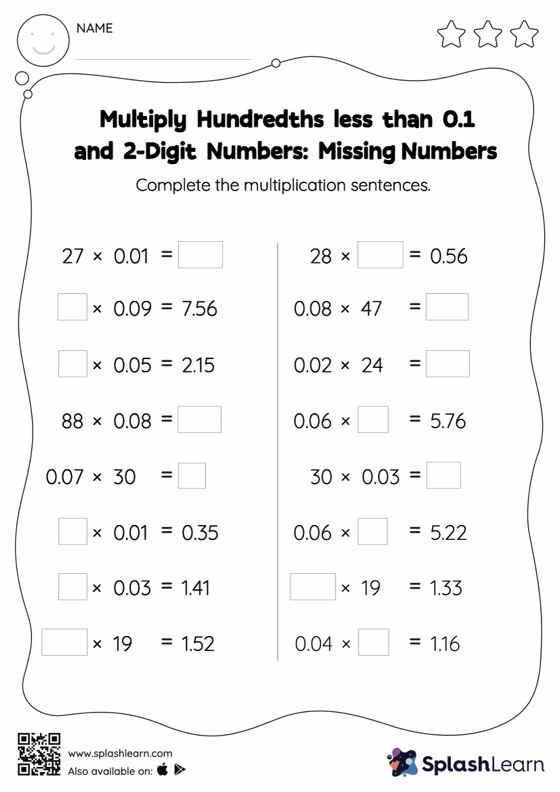# Multiply Hundredths less than 0.1 and 2-Digit Numbers: Missing Numbers Worksheet

Home > Multiply Hundredths less than 0.1 and 2-Digit Numbers: Missing NumbersLearners hone their math skills with this multiply hundredths less than 0.1 And 2-digit numbers worksheet. Students find the missing number in decimal multiplication in multiply hundredths less than 0.1 And 2-digit numbers worksheet. They may omit the decimal point when multiplying a decimal by a two-digit number and then add it when writing the answer. In each problem, the numbers are laid out in the horizontal format. Students should try to use different strategies involving composing and decomposing numbers to solve these problems. This will help them develop flexibility and fluency.